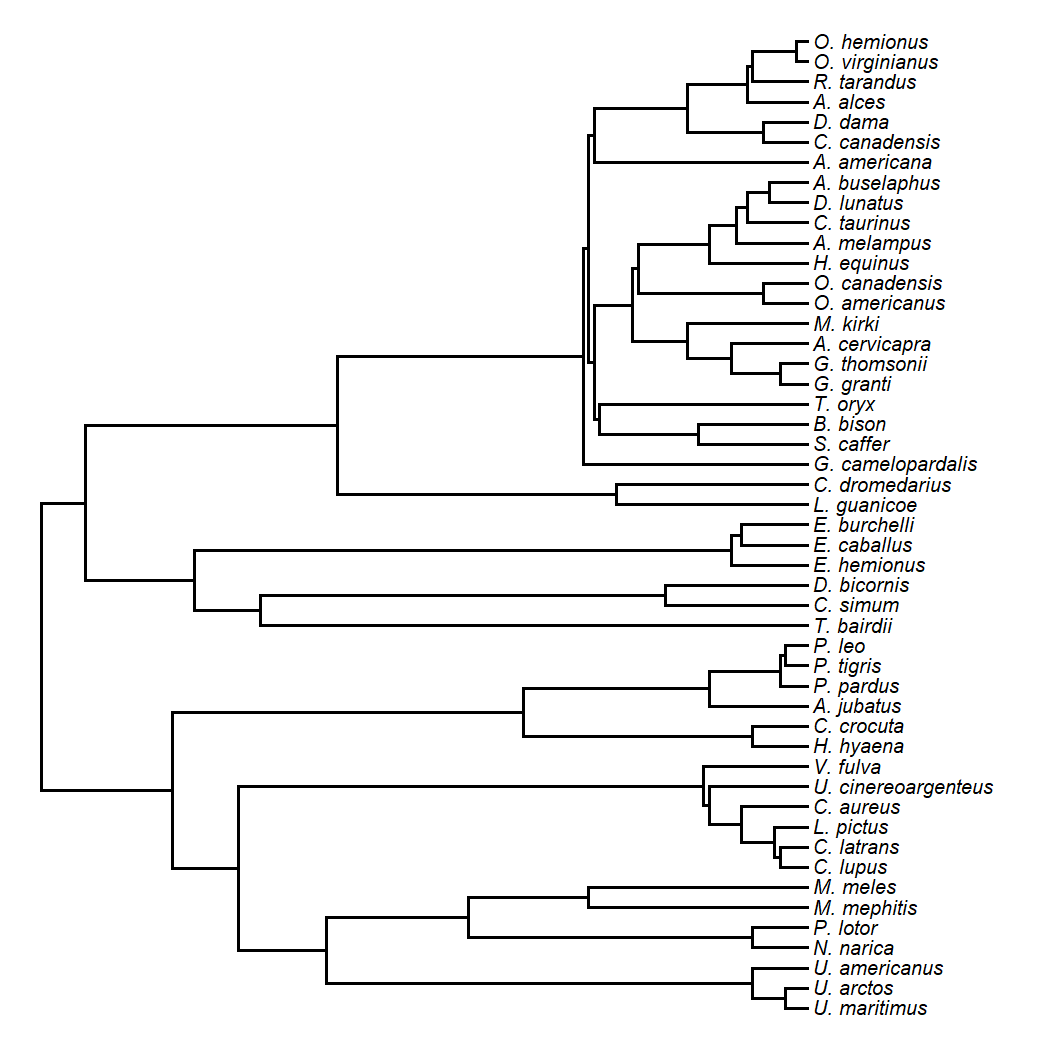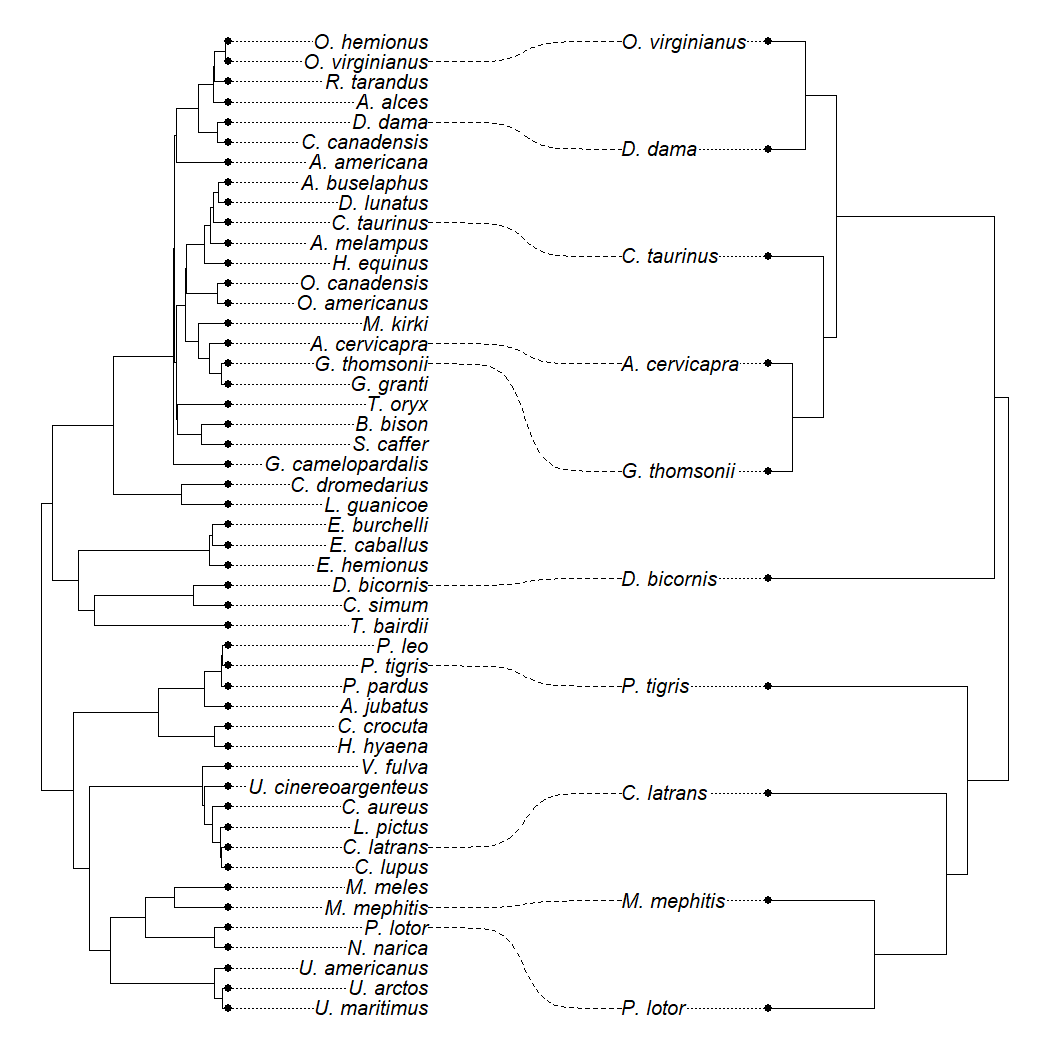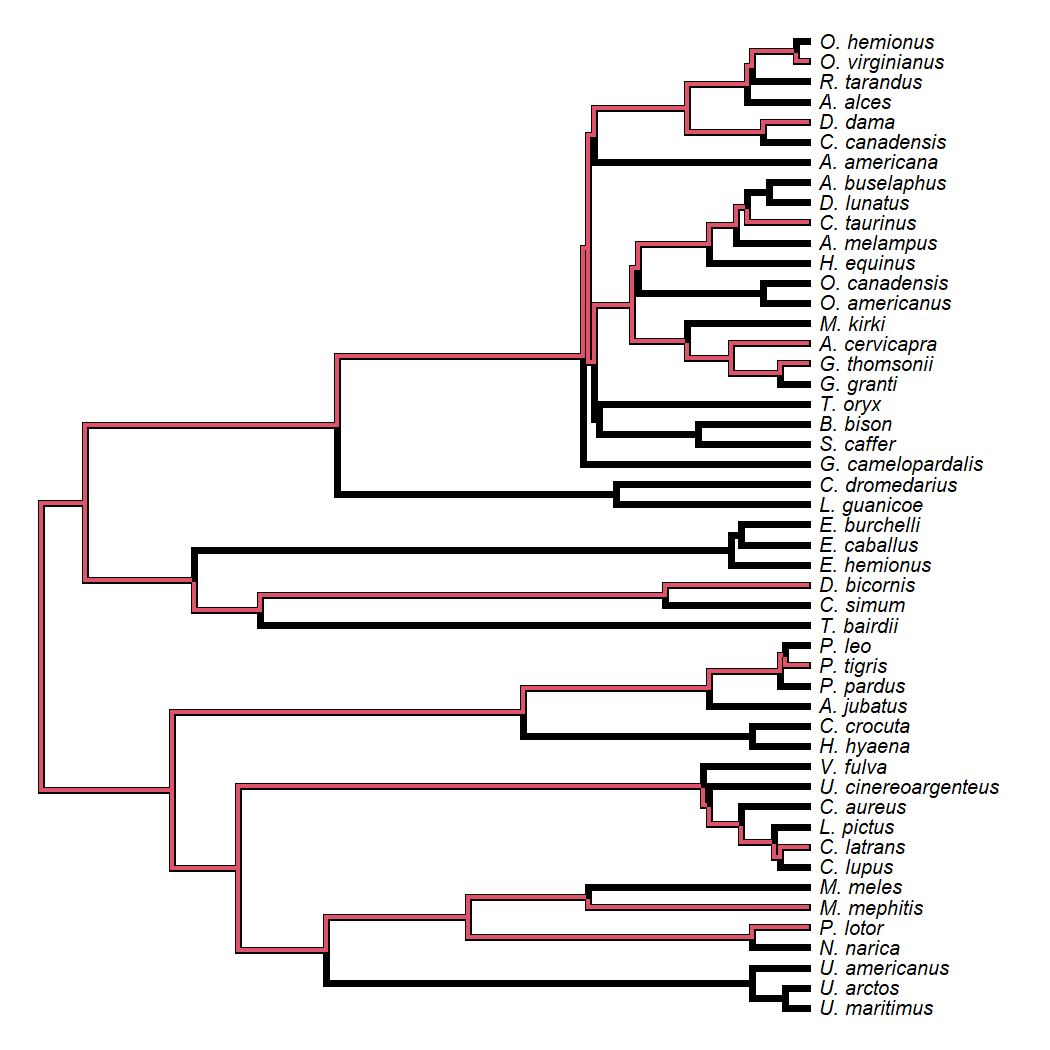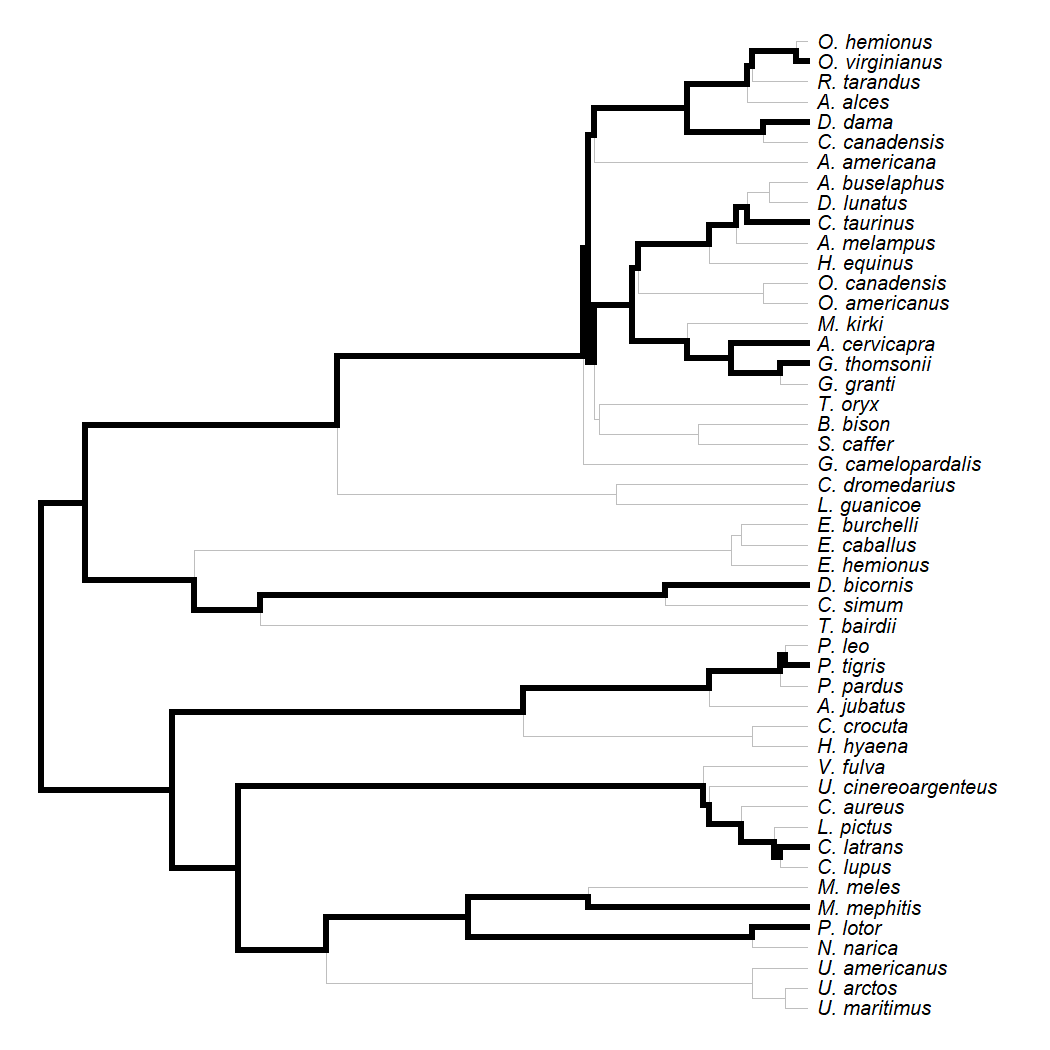## Tuesday, February 23, 2021

### Overplotting a tree of sampled species on a fully-sampled tree

Today a friend & colleague asked me for advice on how, given a full tree & a pruned tree (representing, for example, the subset of species for which comparative data had been obtained) overplot the pruned tree (in color) on the full tree. The goal would be to illustrate (heuristically) the phylogenetic coverage of a sample (e.g., are we missing major clades?; are clades sampled unevenly?).

This can be done. Here's an illustration.

To start, let's get a tree to work with. I'll use this phylogeny for mammals:

``````library(phytools)
data(mammal.tree)
plotTree(mammal.tree,ftype="i",fsize=0.8)
``````Now let's imagine we have a random subset of these taxa, as follows:

``````our.mammals<-sample(mammal.tree\$tip.label,10)
our.mammals
``````
``````##   "D._dama"        "C._latrans"     "C._taurinus"    "G._thomsonii"
##   "M._mephitis"    "D._bicornis"    "O._virginianus" "P._lotor"
##   "A._cervicapra"  "P._tigris"
``````

Here's our pruned tree:

``````pruned.mammals<-keep.tip(mammal.tree,our.mammals)
plot(cophylo(mammal.tree,pruned.mammals,rotate=FALSE),
``````Now let's do the overlaying thing.

For this, I'm going to first identify all the nodes ancestral to our sampled species in the original tree.

``````library(phangorn)
tips<-sapply(our.mammals,function(x,y) which(y==x),
y=mammal.tree\$tip.label)
tips
``````
``````##        D._dama     C._latrans    C._taurinus   G._thomsonii    M._mephitis
##             45              9             40             33              6
##    D._bicornis O._virginianus       P._lotor  A._cervicapra      P._tigris
##             22             48              5             34             18
``````
``````nodes<-lapply(tips,Ancestors,x=mammal.tree)
nodes<-c(tips,unique(unlist(nodes)))
``````

Now I need to remove the root node - because it doesn't correspond to an internal edge.

I also need to remove any nodes that are themselves ancestral to the MRCA of all our sampled species!

I can actually do both things at once as follows:

``````aa<-getMRCA(mammal.tree,our.mammals)
nodes<-setdiff(nodes,c(aa,Ancestors(mammal.tree,aa)))
``````

Finally, I can paint my tree & plot it:

``````painted<-paintBranches(mammal.tree,edge=nodes,state="2")
plotTree(mammal.tree,lwd=5,ftype="i",fsize=0.8)
par(fg="transparent")
ftype="i",fsize=0.8)
````````````## no colors provided. using the following legend:
##         1         2
##   "black" "#DF536B"
``````
``````par(fg="black")
``````

Awesome. That's not perfect, but it's not bad.

If we wanted, we could make the base tree thin & the overplot stronger. This is what that looks like:

``````par(fg="transparent")
plotTree(mammal.tree,lwd=1,ftype="i",fsize=0.8,color="grey")
par(fg="black")
cols<-setNames(c("transparent","black"),1:2)
plot(painted,color=cols,